Checkout JEE MAINS 2022 Question Paper Analysis : Checkout JEE MAINS 2022 Question Paper Analysis :

# Types Of Polygon

Before we start learning about types of polygon, let us first learn the definition of a polygon. A polygon is a closed shape figure that has a minimum of three sides and three vertices. The term ‘poly’ means ‘many’ and ‘gon’ means ‘angle’. Thus, polygons have many angles. The perimeter and area of polygon depend upon its types.

The classification of polygons is described based on the numbers of sides and vertices. For example, a polygon as four sides and four angles, then it is quadrilateral. The polygons up to 12 sides are the important ones. We are going to learn here polygon’s types based on the number of sides of the closed figure along with examples and diagrams.

All the polygon from triangle to decagon are given here in the below figures, respectively.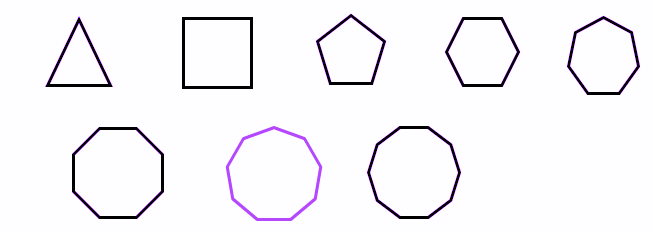These are the basic types of regular polygons which we see in our daily life. Also, these polygons have certain properties based on their area, perimeter, diagonals, etc.

## Classification of Polygons and their Properties

A two-dimensional shape which is enclosed by a finite number of straight lines joining in the form of a closed-loop is called a polygon. The line segments which make the polygon are known as polygon’s sides or edges. Whereas the corner or the point where any two sides join is called the vertex of the polygon. Now, based on the number of sides and angles, polygons are classified into different types, which we are going to discuss here.

Polygons are classified into various types based on the number of sides and measures of the angles. They are:

• Regular Polygons
• Irregular Polygons
• Concave Polygons
• Convex Polygons
• Trigons
• Pentagon Polygons
• Hexagon Polygons
• Equilateral Polygons
• Equiangular Polygons

Let us discuss them one by one.

### Regular Polygon

In a regular polygon, all the sides of the polygon are equal, and all the interior angles are the same. For example, a regular hexagon has six equal sides, and all its interior angles measure to 120 degrees. Few more examples are mentioned below.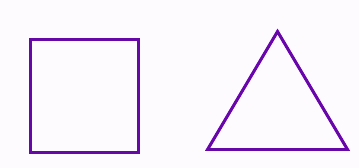Examples:

• A square has all its sides equal to 5cm, and all the angles are at 90°.
• An equilateral triangle has all three sides equal to 10cm and angles measure to 60°.
• A regular pentagon has 5 equal sides and all the interior angles measures to 108 degrees.

### Irregular Polygon

A polygon with an irregular shape. It means the sides and angles of the polygon are not equal.

Example:

• A quadrilateral with unequal sides.
• An isosceles triangle has only two of its sides equal, and the third side has a different measurement.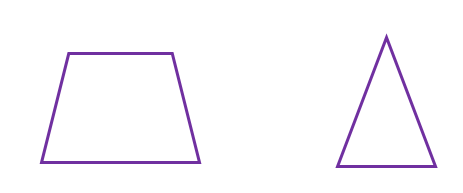### Convex Polygon

In a convex polygon, the measure of the interior angle is less than 180 degrees. It is exactly opposite to the concave polygon. The vertices of a convex polygon are always outwards.

Example: See the figure of an irregular hexagon, whose vertices are outwards.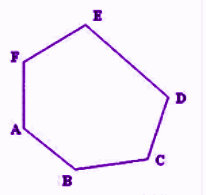### Concave Polygon

In a concave polygon, at least one angle measures more than 180 degrees. The vertices of a concave polygon are inwards as well as outwards.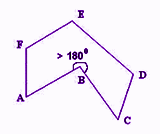### Trigons

Trigons are polygons who have three sides. They are nothing but triangles. These trigons or triangles are further classified into different categories, such as:

• Scalene Triangle: All sides are unequal
• Isosceles Triangle: Two sides are equal
• Equilateral Triangle: All the three sides are equal and all angles measures to 60 degrees.

Quadrilateral polygon is also called a four-sided polygon or a quadrangle. The different types of the quadrilateral polygon are square, rectangle, rhombus and parallelogram.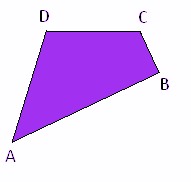### Pentagon Polygon

The five-sided polygon is called pentagon polygon. When all the five sides of the polygon are equal in length, then it is called regular pentagon otherwise irregular pentagon.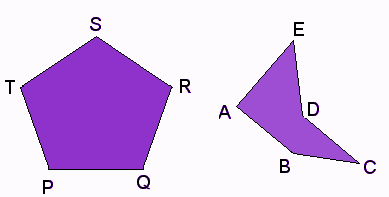### Hexagons

Another type of polygon is the hexagon which has 6 sides and 6 vertices. A regular hexagon will have equal 6 sides and all its interior and exterior angles also measure equals.

### Equilateral Polygons

The polygons whose all the sides are equal are called equilateral polygons, for example, an equilateral triangle, a square, etc.

### Equiangular Polygons

The polygons whose all the interior angles are equal such as a rectangle are called equiangular polygons.

### Types of Polygon With Sides 3 to 20

Now let us discuss, types of regular polygon according to the number of sides and measurement of angles.

 Name of the Polygons Sides Vertices Angle Triangle (also called Trigon) 3 3 60° Quadrilateral (also called Tetragon) 4 4 90° Pentagon 5 5 108° Hexagon 6 6 120° Heptagon 7 7 128.571° Octagon 8 8 135° Nonagon (also called Enneagon) 9 9 140° Decagon 10 10 144° Hendecagon 11 11 147.27 Dodecagon 12 12 150 Tridecagon or triskaidecagon 13 13 152.3 Tetradecagon or tetrakaidecago 14 14 154.28 Pendedecagon 15 15 156 Hexdecagon 16 16 157.5 Heptdecagon 17 17 158.82 Octdecagon 18 18 160 Enneadecagon 19 19 161.05 Icosagon 20 20 162 n-gon n n (n-2)× 180° / n

Test your Knowledge on Types Of Polygon